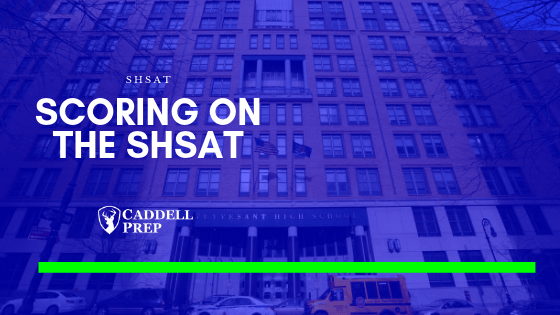# Scoring on the SHSAT

In this video we will take a look at how the scaled score is determined on the SHSAT and how to account for that when preparing for the SHSAT. Want to convert your score? Try our SHSAT Score Calculator.

## Video Transcript

The way scoring works on the SHSAT is that they score the ELA section and the Math section separately then add it together.

Each section is worth up to 400 points and the highest score you can get in SHSAT is 800, theoretically.

However, each year the highest score is in the low 700s.

When each section is scored, each section is scored based on a normal curve.

It gets a little tricky so let’s look at an example.

Here, we have the old scoring table.

For example, if you had 10 out of 50 questions right, that’s 20% and the scale score is 129.Something worth noting is that the difference between 1 and 2 is not the same as the difference between getting 15 and 16 right.

If you go from one correct answer to two correct answers, that increases your score by 14 points. Whereas, if you go from 15 to 16, still one more question correct, it only increases your score by 6 points. And then you’ll see, as we go towards the middle, it starts to increase by about 4 points.

In the middle area, each correct answer increases by 4 points.

Bu then, it changes again when we go out in the middle.

As we go from 40 to 41, it increases to 5 points. From 45 to 46, it increases by 10 points. And then from 47 to 48, that’s 11 points. It gets really drastic.

My point is if you increase your score at the high-end for one of these tests, you’re going to increase your overall score significantly.

These are the 2017 cutoff scores for a few schools and let’s look at an example.

Let’s say overall you got a 68%. For ELA you got 68% and for Math is 68%. You’ll have 246 on both sections. If we add that up, that’s a score of 492. You could have made it in Brooklyn Tech but not in Staten Island Tech and Stuyvesant.

But let’s say you still have 68% overall score. But on one section you got 50% correct and on the other section you got 86% correct. So, you did really well on one and not so good on the other but overall the average is 68%.

If we look at this, 50% corresponds to 210 and 86% is 290. When we add these up, we got a 500. The same overall score but the scale increased by 8 points.

The scale didn’t change so the student still didn’t make it in Staten Island Tech or Stuyvesant. But if we look at a more drastic one, we will see that it would make a difference.

But let’s say we’re aiming for a higher score. It’s more noticeable if we look at greater scores.

Lest’s say overall it’s 70%. Let’s see if we have 70 on both sections and see what that gives us. So, 70 and 70 that would be 250 and 250. Altogether that’s 500. So, it’s the same as what we got in 68%.

Let’s look at a more skewed one. In this case, we get a 50% on one section and a 90% in the other section. 50 that would be 210 but then for 90% that’s 308. Altogether it’s 518.

That would have made it into Staten Island Tech for 2017. A 50 on one section and a 90 on the other.

Now, of course, you don’t have to get a 50 right? You can improve this also and then improving that will increase your chances of getting to a more selective school such as Stuyvesant.